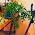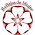### MATH 1280 Introduction to Statistics – Assignment Unit 4 – Part 3

Evening of summer, painted by Halfdan Johnsen Egedius

A Population:
19. Determine how many observations in the pop3.csv file are of type a: 33,333.

R code:
> counts<-data.frame(table(pop3))
> counts

20. Using the appropriate R function with the defaul options, what is the median of the time column of pop3 (round to 3 decimal places): 4.473

R Code:
> round(median(pop3\$time), 3)
 4.473

Portrait of Mari Clasen, painted by Halfdan Johnsen Egedius

21. What is the variance of the time column of pop3 (rounded to three decimal places)?

R Code:
> round(var(pop3\$time), 3)

 54.916

Halfdan Johnsen Egedius was born in Norway in 1877

1.Beautiful art.

1.Thank you to visit:
MATH 1280 Introduction to Statistics – Assignment Unit 4 – Part 3
-
A Population:
19. Determine how many observations in the pop3.csv file are of type a: 33,333.

R code:
> counts<-data.frame(table(pop3))
> counts

2.Interesting post! thanks for sharing!
Don't Call Me Fashion Blogger
Bloglovin'

1.Thank you to visit:
MATH 1280 Introduction to Statistics – Assignment Unit 4 – Part 3
-

3.1.Thank you to visit:
MATH 1280 Introduction to Statistics – Assignment Unit 4 – Part 3
-
20. Using the appropriate R function with the defaul options, what is the median of the time column of pop3 (round to 3 decimal places): 4.473

R Code:
> round(median(pop3\$time), 3)
 4.473

4.Because I don't understand the math, I'll comment on the paintings...

I had to look up Egedius. Such a tragically short life! Only 21 when he died. The work is interesting - a rather harsh realism.

1.Thank you to visit:
MATH 1280 Introduction to Statistics – Assignment Unit 4 – Part 3
-
21. What is the variance of the time column of pop3 (rounded to three decimal places)?

R Code:

> round(var(pop3\$time), 3)

 54.916

5.Ilove maths but I'm afraid I forgot many things!!
xoxo

marisasclosetblog.com

1.Thank you to visit:
MATH 1280 Introduction to Statistics – Assignment Unit 4 – Part 3
-
A Population:
19. Determine how many observations in the pop3.csv file are of type a: 33,333.

R code:
> counts<-data.frame(table(pop3))
> counts

6.I love the paintings, too:) Math has always been quite challenging for me:(

1.Thank you to visit:
MATH 1280 Introduction to Statistics – Assignment Unit 4 – Part 3
-
20. Using the appropriate R function with the defaul options, what is the median of the time column of pop3 (round to 3 decimal places): 4.473

R Code:
> round(median(pop3\$time), 3)
 4.473

7.The first painting is stunning!☺

1.Thank you to visit:
MATH 1280 Introduction to Statistics – Assignment Unit 4 – Part 3
-
21. What is the variance of the time column of pop3 (rounded to three decimal places)?

R Code:
> round(var(pop3\$time), 3)
 54.916

8.The artwork of Egedius is gorgeous! The math goes over my head. :)

1.Thank you to visit:
MATH 1280 Introduction to Statistics – Assignment Unit 4 – Part 3
-
A Population:
19. Determine how many observations in the pop3.csv file are of type a: 33,333.

R code:
> counts<-data.frame(table(pop3))
> counts

9.interesting information and images ☺
best regards
Lili

1.Thank you to visit:
MATH 1280 Introduction to Statistics – Assignment Unit 4 – Part 3
-
20. Using the appropriate R function with the defaul options, what is the median of the time column of pop3 (round to 3 decimal places): 4.473

R Code:
> round(median(pop3\$time), 3)
 4.473

10.great, I like maths

1.Thank you to visit:
MATH 1280 Introduction to Statistics – Assignment Unit 4 – Part 3
-
21. What is the variance of the time column of pop3 (rounded to three decimal places)?